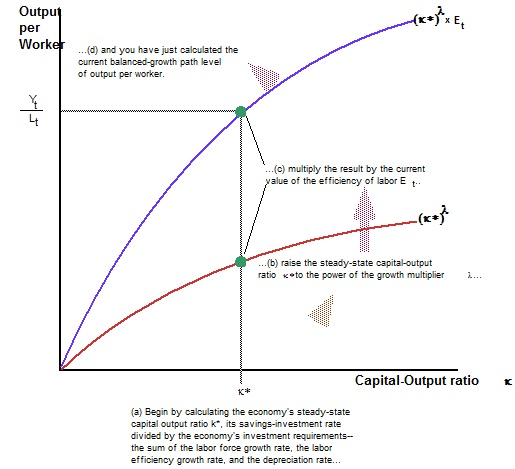#### Forecasting the Economy’s Destiny and calculating balanced growth path

Forecasting the Economy’s Destiny:

The typical Solow growth model makes estimating an economy’s long-run progress destiny normal:

a) Compute the steadystate capital output ratio, k*=s/(n+g+δ), equivalent to the savings share divided by investment requirements.

b) Increase the steady state capital output ratio k* by increasing it to power of growth multiplier λ = (α/(1-α)), where α is production function’s diminishing returns to scale parameter.

c) Multiply result by the present efficiency of labor Et.

You have just calculated output for each worker on economy’s balanced-growth path. If you just wish to understand the present, you are done. If you wish to also estimate the future, then:

Estimate that balanced growth output for each worker will increase at similar  proportional rate g as labour efficiency.

If economy is on its balancedgrowth path, you are done. Except if economy isn’t currently on its balanced growth path, then:

a) Estimate that the economy is heading for its balanced-growth path.
b) Estimate that the economy will grow along its balanced-growth path after it has converged to it.

The growth model makes forecasts of the long-run destiny of the economy straightforward, and provides an easy way to analyze how the factors making for (a) higher capital intensity and (b) better technology and labor efficiency determine output per worker.

How to Calculate the Economy’s Balanced-Growth Path:Calculating the balanced-growth level of output per worker is simple: (i) calculate the steady-state capital-output ratio k* (ii) raise k* to the power of the growth multiplier λ, and (iii) multiply the result by the efficiency of labor Et.

What happens if there is a change in economic policy or in the economic environment—if something happens to change s, the savings rate; n, the labor force growth rate; g, the efficiency of labor growth rate; or δ, the depreciation rate? If any of these change, the economy’s steady-state balanced-growth path changes as well. So calculate the new steady-state balanced-growth path, and then forecast that the economy will converge to this new balanced-growth path.

Why, and how, does this growth model work? Why is there a steady-state growth path? Why do these calculations above tell us what it is? To understand these issues, we need to back up and dig a little deeper.

Latest technology based Macroeconomics Online Tutoring Assistance

Tutors, at the www.tutorsglobe.com, take pledge to provide full satisfaction and assurance in Macroeconomics help via online tutoring. Students are getting 100% satisfaction by online tutors across the globe. Here you can get homework help for Macroeconomics, project ideas and tutorials. We provide email based Macroeconomics help. You can join us to ask queries 24x7 with live, experienced and qualified online tutors specialized in Macroeconomics. Through Online Tutoring, you would be able to complete your homework or assignments at your home. Tutors at the TutorsGlobe are committed to provide the best quality online tutoring assistance for Macroeconomics Homework help and assignment help services. They use their experience, as they have solved thousands of the Macroeconomics assignments, which may help you to solve your complex issues of Macroeconomics. TutorsGlobe assure for the best quality compliance to your homework. Compromise with quality is not in our dictionary. If we feel that we are not able to provide the homework help as per the deadline or given instruction by the student, we refund the money of the student without any delay.